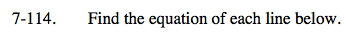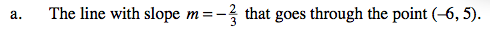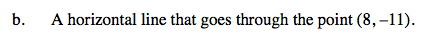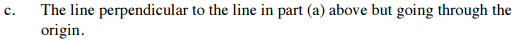### Home > AC > Chapter 7 > Lesson 7.3.4 > Problem7-114

7-114.Using y = mx + b, substitute the values of m, y, and x and solve for b.

Solve for b and rewrite the equation substituting m and b back into their original positions.A horizontal line has zero slope. Therefore, simply substitute the values for x and y into the equation and solve for b.
Then, rewrite the equation with m and b substituted back into their original equations.

y = −11See parts (a) and (b) to solve. Remember that perpendicular lines have opposite reciprocal slopes.